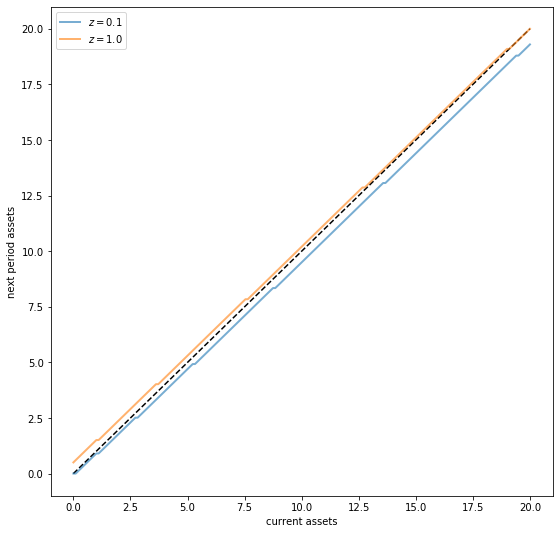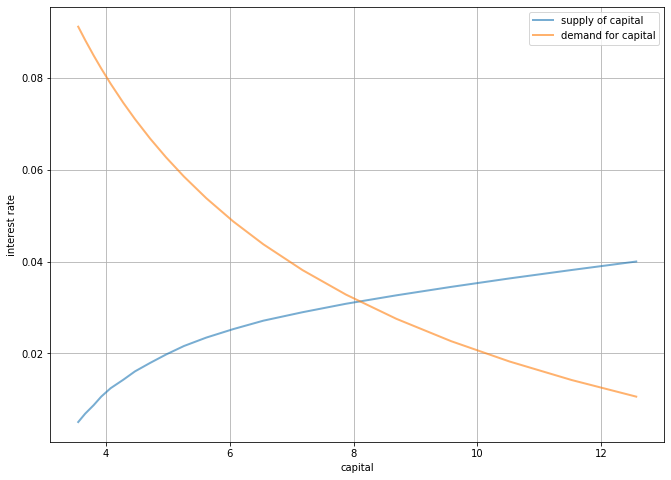# The Aiyagari Model¶

## Contents¶

In addition to what’s in Anaconda, this lecture will need the following libraries:

In :
!pip install quantecon


## Overview¶

In this lecture, we describe the structure of a class of models that build on work by Truman Bewley [Bew77].

We begin by discussing an example of a Bewley model due to Rao Aiyagari.

The model features

• Heterogeneous agents
• A single exogenous vehicle for borrowing and lending
• Limits on amounts individual agents may borrow

The Aiyagari model has been used to investigate many topics, including

• precautionary savings and the effect of liquidity constraints [Aiy94]
• risk sharing and asset pricing [HL96]
• the shape of the wealth distribution [BBZ15]
• etc., etc., etc.

Let’s start with some imports:

In :
import numpy as np
import quantecon as qe
import matplotlib.pyplot as plt
%matplotlib inline
from quantecon.markov import DiscreteDP
from numba import jit

/home/ubuntu/anaconda3/lib/python3.7/site-packages/numba/np/ufunc/parallel.py:355: NumbaWarning: The TBB threading layer requires TBB version 2019.5 or later i.e., TBB_INTERFACE_VERSION >= 11005. Found TBB_INTERFACE_VERSION = 11004. The TBB threading layer is disabled.
warnings.warn(problem)


The primary reference for this lecture is [Aiy94].

A textbook treatment is available in chapter 18 of [LS18].

A continuous time version of the model by SeHyoun Ahn and Benjamin Moll can be found here.

## The Economy¶

### Households¶

Infinitely lived households / consumers face idiosyncratic income shocks.

A unit interval of ex-ante identical households face a common borrowing constraint.

The savings problem faced by a typical household is

$$\max \mathbb E \sum_{t=0}^{\infty} \beta^t u(c_t)$$

subject to

$$a_{t+1} + c_t \leq w z_t + (1 + r) a_t \quad c_t \geq 0, \quad \text{and} \quad a_t \geq -B$$

where

• $c_t$ is current consumption
• $a_t$ is assets
• $z_t$ is an exogenous component of labor income capturing stochastic unemployment risk, etc.
• $w$ is a wage rate
• $r$ is a net interest rate
• $B$ is the maximum amount that the agent is allowed to borrow

The exogenous process $\{z_t\}$ follows a finite state Markov chain with given stochastic matrix $P$.

The wage and interest rate are fixed over time.

In this simple version of the model, households supply labor inelastically because they do not value leisure.

## Firms¶

Firms produce output by hiring capital and labor.

Firms act competitively and face constant returns to scale.

Since returns to scale are constant the number of firms does not matter.

Hence we can consider a single (but nonetheless competitive) representative firm.

The firm’s output is

$$Y_t = A K_t^{\alpha} N^{1 - \alpha}$$

where

• $A$ and $\alpha$ are parameters with $A > 0$ and $\alpha \in (0, 1)$
• $K_t$ is aggregate capital
• $N$ is total labor supply (which is constant in this simple version of the model)

The firm’s problem is

$$max_{K, N} \left\{ A K_t^{\alpha} N^{1 - \alpha} - (r + \delta) K - w N \right\}$$

The parameter $\delta$ is the depreciation rate.

From the first-order condition with respect to capital, the firm’s inverse demand for capital is

$$r = A \alpha \left( \frac{N}{K} \right)^{1 - \alpha} - \delta \tag{1}$$

Using this expression and the firm’s first-order condition for labor, we can pin down the equilibrium wage rate as a function of $r$ as

$$w(r) = A (1 - \alpha) (A \alpha / (r + \delta))^{\alpha / (1 - \alpha)} \tag{2}$$

### Equilibrium¶

We construct a stationary rational expectations equilibrium (SREE).

In such an equilibrium

• prices induce behavior that generates aggregate quantities consistent with the prices
• aggregate quantities and prices are constant over time

In more detail, an SREE lists a set of prices, savings and production policies such that

• households want to choose the specified savings policies taking the prices as given
• firms maximize profits taking the same prices as given
• the resulting aggregate quantities are consistent with the prices; in particular, the demand for capital equals the supply
• aggregate quantities (defined as cross-sectional averages) are constant

In practice, once parameter values are set, we can check for an SREE by the following steps

1. pick a proposed quantity $K$ for aggregate capital
2. determine corresponding prices, with interest rate $r$ determined by (1) and a wage rate $w(r)$ as given in (2)
3. determine the common optimal savings policy of the households given these prices
4. compute aggregate capital as the mean of steady state capital given this savings policy

If this final quantity agrees with $K$ then we have a SREE.

## Code¶

Let’s look at how we might compute such an equilibrium in practice.

To solve the household’s dynamic programming problem we’ll use the DiscreteDP class from QuantEcon.py.

Our first task is the least exciting one: write code that maps parameters for a household problem into the R and Q matrices needed to generate an instance of DiscreteDP.

Below is a piece of boilerplate code that does just this.

In reading the code, the following information will be helpful

• R needs to be a matrix where R[s, a] is the reward at state s under action a.
• Q needs to be a three-dimensional array where Q[s, a, s'] is the probability of transitioning to state s' when the current state is s and the current action is a.

(A more detailed discussion of DiscreteDP is available in the Discrete State Dynamic Programming lecture in the Advanced Quantitative Economics with Python lecture series.)

Here we take the state to be $s_t := (a_t, z_t)$, where $a_t$ is assets and $z_t$ is the shock.

The action is the choice of next period asset level $a_{t+1}$.

We use Numba to speed up the loops so we can update the matrices efficiently when the parameters change.

The class also includes a default set of parameters that we’ll adopt unless otherwise specified.

In :
class Household:
"""
This class takes the parameters that define a household asset accumulation
problem and computes the corresponding reward and transition matrices R
and Q required to generate an instance of DiscreteDP, and thereby solve
for the optimal policy.

Comments on indexing: We need to enumerate the state space S as a sequence
S = {0, ..., n}.  To this end, (a_i, z_i) index pairs are mapped to s_i
indices according to the rule

s_i = a_i * z_size + z_i

To invert this map, use

a_i = s_i // z_size  (integer division)
z_i = s_i % z_size

"""

def __init__(self,
r=0.01,                      # Interest rate
w=1.0,                       # Wages
β=0.96,                      # Discount factor
a_min=1e-10,
Π=[[0.9, 0.1], [0.1, 0.9]],  # Markov chain
z_vals=[0.1, 1.0],           # Exogenous states
a_max=18,
a_size=200):

# Store values, set up grids over a and z
self.r, self.w, self.β = r, w, β
self.a_min, self.a_max, self.a_size = a_min, a_max, a_size

self.Π = np.asarray(Π)
self.z_vals = np.asarray(z_vals)
self.z_size = len(z_vals)

self.a_vals = np.linspace(a_min, a_max, a_size)
self.n = a_size * self.z_size

# Build the array Q
self.Q = np.zeros((self.n, a_size, self.n))
self.build_Q()

# Build the array R
self.R = np.empty((self.n, a_size))
self.build_R()

def set_prices(self, r, w):
"""
Use this method to reset prices. Calling the method will trigger a
re-build of R.
"""
self.r, self.w = r, w
self.build_R()

def build_Q(self):
populate_Q(self.Q, self.a_size, self.z_size, self.Π)

def build_R(self):
self.R.fill(-np.inf)
populate_R(self.R,
self.a_size,
self.z_size,
self.a_vals,
self.z_vals,
self.r,
self.w)

# Do the hard work using JIT-ed functions

@jit(nopython=True)
def populate_R(R, a_size, z_size, a_vals, z_vals, r, w):
n = a_size * z_size
for s_i in range(n):
a_i = s_i // z_size
z_i = s_i % z_size
a = a_vals[a_i]
z = z_vals[z_i]
for new_a_i in range(a_size):
a_new = a_vals[new_a_i]
c = w * z + (1 + r) * a - a_new
if c > 0:
R[s_i, new_a_i] = np.log(c)  # Utility

@jit(nopython=True)
def populate_Q(Q, a_size, z_size, Π):
n = a_size * z_size
for s_i in range(n):
z_i = s_i % z_size
for a_i in range(a_size):
for next_z_i in range(z_size):
Q[s_i, a_i, a_i*z_size + next_z_i] = Π[z_i, next_z_i]

@jit(nopython=True)
def asset_marginal(s_probs, a_size, z_size):
a_probs = np.zeros(a_size)
for a_i in range(a_size):
for z_i in range(z_size):
a_probs[a_i] += s_probs[a_i*z_size + z_i]
return a_probs


As a first example of what we can do, let’s compute and plot an optimal accumulation policy at fixed prices.

In :
# Example prices
r = 0.03
w = 0.956

# Create an instance of Household
am = Household(a_max=20, r=r, w=w)

# Use the instance to build a discrete dynamic program
am_ddp = DiscreteDP(am.R, am.Q, am.β)

# Solve using policy function iteration
results = am_ddp.solve(method='policy_iteration')

# Simplify names
z_size, a_size = am.z_size, am.a_size
z_vals, a_vals = am.z_vals, am.a_vals
n = a_size * z_size

# Get all optimal actions across the set of a indices with z fixed in each row
a_star = np.empty((z_size, a_size))
for s_i in range(n):
a_i = s_i // z_size
z_i = s_i % z_size
a_star[z_i, a_i] = a_vals[results.sigma[s_i]]

fig, ax = plt.subplots(figsize=(9, 9))
ax.plot(a_vals, a_vals, 'k--')  # 45 degrees
for i in range(z_size):
lb = f'$z = {z_vals[i]:.2}$'
ax.plot(a_vals, a_star[i, :], lw=2, alpha=0.6, label=lb)
ax.set_xlabel('current assets')
ax.set_ylabel('next period assets')
ax.legend(loc='upper left')

plt.show()The plot shows asset accumulation policies at different values of the exogenous state.

Now we want to calculate the equilibrium.

Let’s do this visually as a first pass.

The following code draws aggregate supply and demand curves.

The intersection gives equilibrium interest rates and capital.

In :
A = 1.0
N = 1.0
α = 0.33
β = 0.96
δ = 0.05

def r_to_w(r):
"""
Equilibrium wages associated with a given interest rate r.
"""
return A * (1 - α) * (A * α / (r + δ))**(α / (1 - α))

def rd(K):
"""
Inverse demand curve for capital.  The interest rate associated with a
given demand for capital K.
"""
return A * α * (N / K)**(1 - α) - δ

def prices_to_capital_stock(am, r):
"""
Map prices to the induced level of capital stock.

Parameters:
----------

am : Household
An instance of an aiyagari_household.Household
r : float
The interest rate
"""
w = r_to_w(r)
am.set_prices(r, w)
aiyagari_ddp = DiscreteDP(am.R, am.Q, β)
# Compute the optimal policy
results = aiyagari_ddp.solve(method='policy_iteration')
# Compute the stationary distribution
stationary_probs = results.mc.stationary_distributions
# Extract the marginal distribution for assets
asset_probs = asset_marginal(stationary_probs, am.a_size, am.z_size)
# Return K
return np.sum(asset_probs * am.a_vals)

# Create an instance of Household
am = Household(a_max=20)

# Use the instance to build a discrete dynamic program
am_ddp = DiscreteDP(am.R, am.Q, am.β)

# Create a grid of r values at which to compute demand and supply of capital
num_points = 20
r_vals = np.linspace(0.005, 0.04, num_points)

# Compute supply of capital
k_vals = np.empty(num_points)
for i, r in enumerate(r_vals):
k_vals[i] = prices_to_capital_stock(am, r)

# Plot against demand for capital by firms
fig, ax = plt.subplots(figsize=(11, 8))
ax.plot(k_vals, r_vals, lw=2, alpha=0.6, label='supply of capital')
ax.plot(k_vals, rd(k_vals), lw=2, alpha=0.6, label='demand for capital')
ax.grid()
ax.set_xlabel('capital')
ax.set_ylabel('interest rate')
ax.legend(loc='upper right')

plt.show()• Share page